"Math and Multiplication games" App Analytics: ASO Keyword Monitoring | ASOTools

# "Math and Multiplication games" App Analytics: ASO Keyword Monitoring | ASOTools

Are you doing Math and Multiplication games app analytics for better app store ASO strategies? Whether Math and Multiplication games in app store keep on top? What keyword tactics Math and Multiplication games take to rank well in app store? Are app store keywords like "math planet lite", "multiplicand", "restas y sumas" matter in Math and Multiplication games ranking?

Free ASO tools for Google Play & App Store, ASOTools will reveal the app store keywords secrets of Math and Multiplication games.

## App Analytics: Math and Multiplication games

Math and Multiplication games-Fun 3rd grade math games, published by Aleksandr Kutenkov in app store, released on 2020-06-30, and last updated 2021-04-04. It belongs to all category, and all category is ranking no rank in app store. Currently, Math and Multiplication games has 614 ratings, the average rating is 4. Additionally, it had - downloads and - revenue last month in app store worldwide, and the daily active users is -.

More Math and Multiplication games info and other app analytics can be found freely in ASOTool.

## ASO Keyword Monitoring: Math and Multiplication games

### 1.Math and Multiplication games Top 10 App Store Keywords

 Keyword Search Volume KD Related Apps Math and Multiplication games's Rank math planet lite 1 426 12 62 1 multiplicand - - 12 103 1 restas y sumas - - 12 115 1 juegos de restas y sumas para niños - - 13 28 1 math planet pro - - 12 62 1 juego de tablas de multiplicar gratis para niños 2 - 11 46 1 multiplication games math free - - 39 234 1 math games for 3rd graders free multiplication and - - 12 37 1 juegos de tablas de multiplicar gratis para niños 2 - 11 46 1 juegos de tablas de multiplicar gratis - - 10 101 1

App store keywords matter for ASO. If your app is similar to Math and Multiplication games, when you optimize app store keyword ranking, pay attention to the metrics above. ASOTools will show you all of them for free.

Obviously, Math and Multiplication games is ranking for "math planet lite", "multiplicand", "restas y sumas", "juegos de restas y sumas para niños", "math planet pro", "juego de tablas de multiplicar gratis para niños", "multiplication games math free", "math games for 3rd graders free multiplication and", "juegos de tablas de multiplicar gratis para niños", and "juegos de tablas de multiplicar gratis" these top 10 keywords in app store.

Also, you'll find Math and Multiplication games's position in app store search result. Math and Multiplication games is ranking 1 for math planet lite, ranking 1 for multiplicand, ranking 1 for restas y sumas, and ranking 1 for juegos de restas y sumas para niños. For more Math and Multiplication games keyword monitoring, please visit asotools.io.

### 2. Top 5 Keyword-Related Apps& App Analytics

• math planet lite  Related Apps & App Analytics
 Apps Average Star Ratings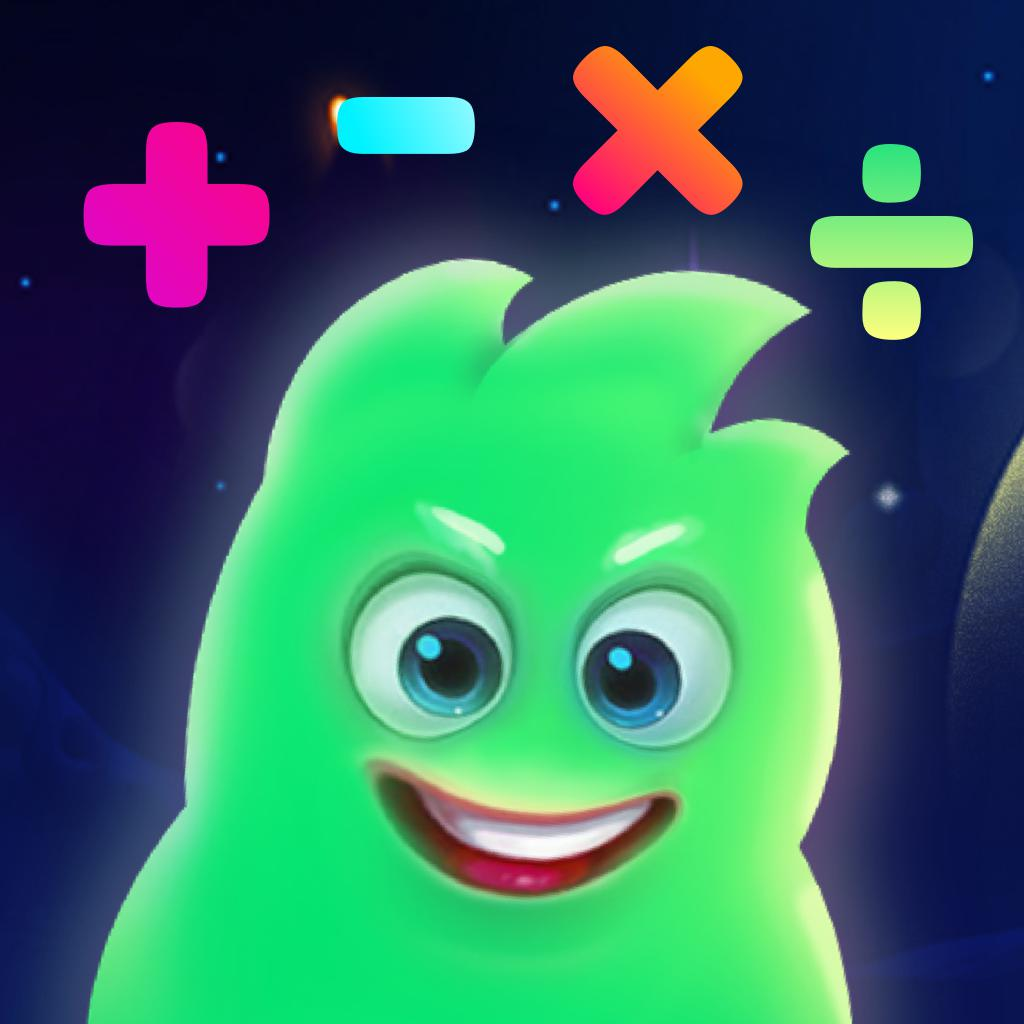Math and Multiplication games-Fun 3rd grade math games 4 614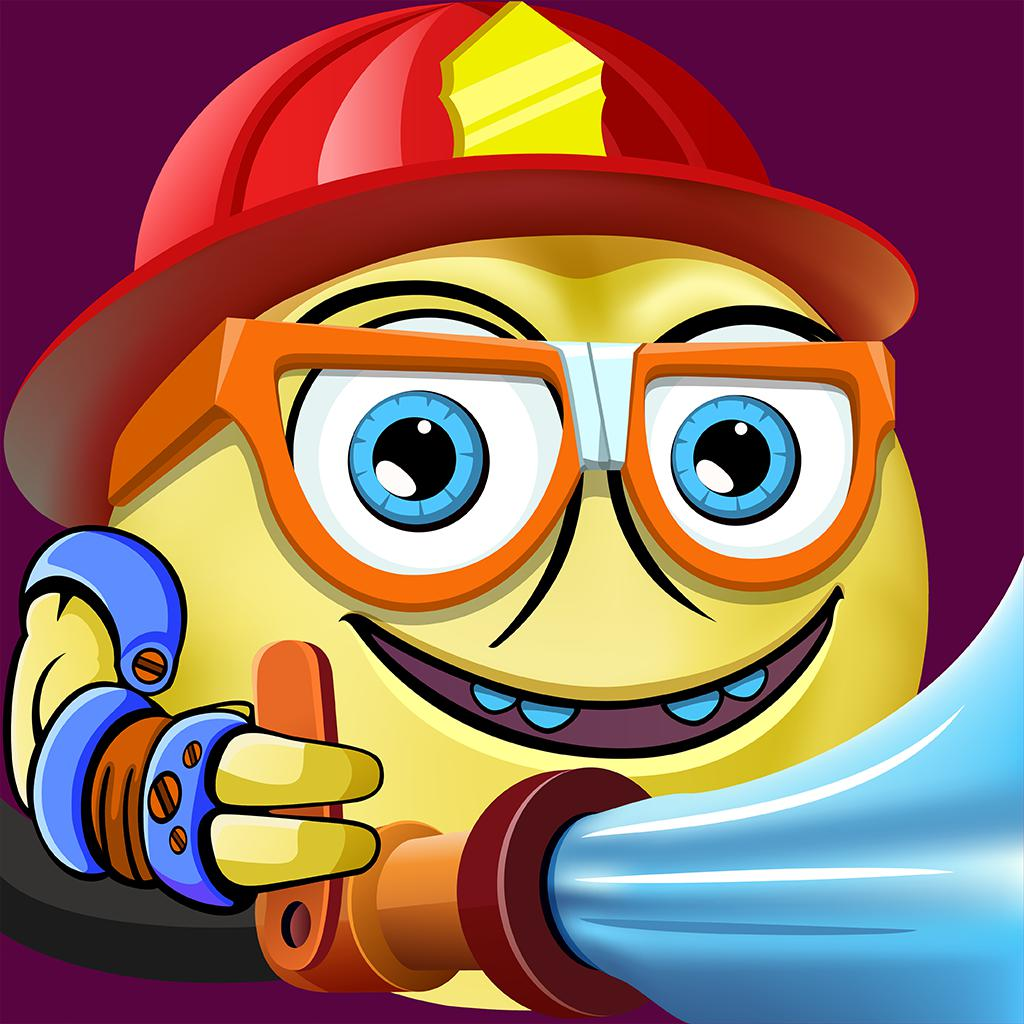Math Rescue: 7－9 Year Old Game-Boys & Girl Educational Games 4 477Kids Learning Puzzles: Dogs, My Math Educreations 5 1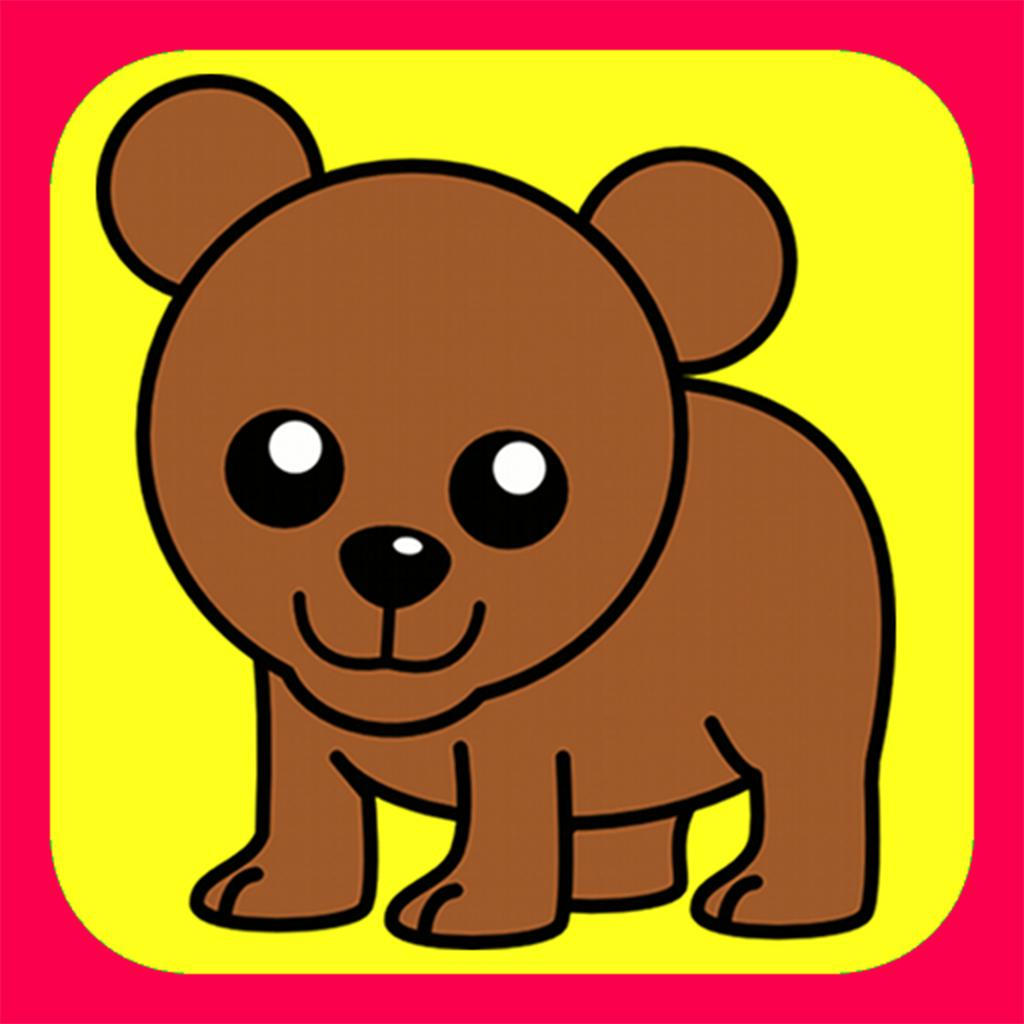Animal Fun Facts 5 8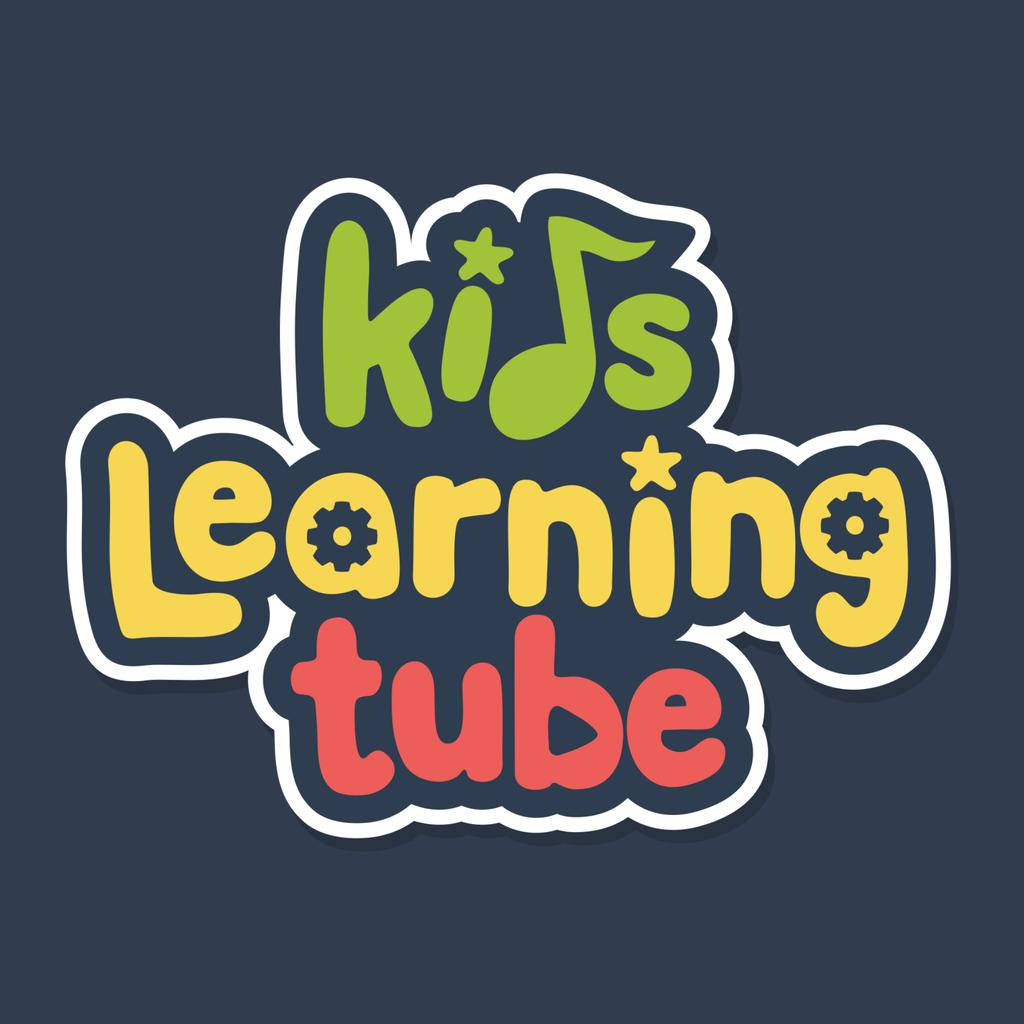Kids Learning Tube-Educating kids of all ages 4 308

"math planet lite" has 62 apps. Top 5 are Math and Multiplication games, Math Rescue: 7－9 Year Old Game, Kids Learning Puzzles: Dogs, My Math Educreations, Animal Fun Facts, and Kids Learning Tube.

• multiplicand  Related Apps & App Analytics
 Apps Average Star RatingsMath and Multiplication games-Fun 3rd grade math games 4 614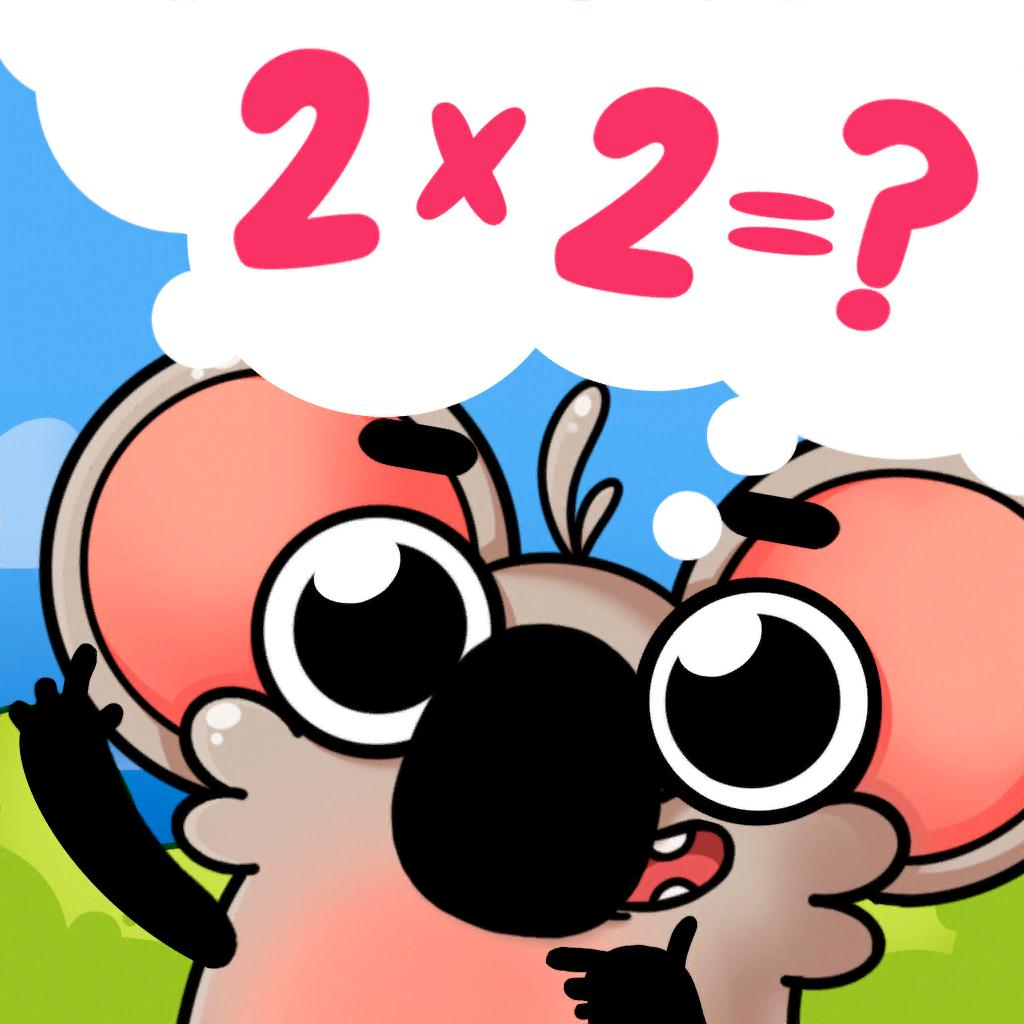Multiplication Games For Kids.-Engaging Times Tables (Game) 4 687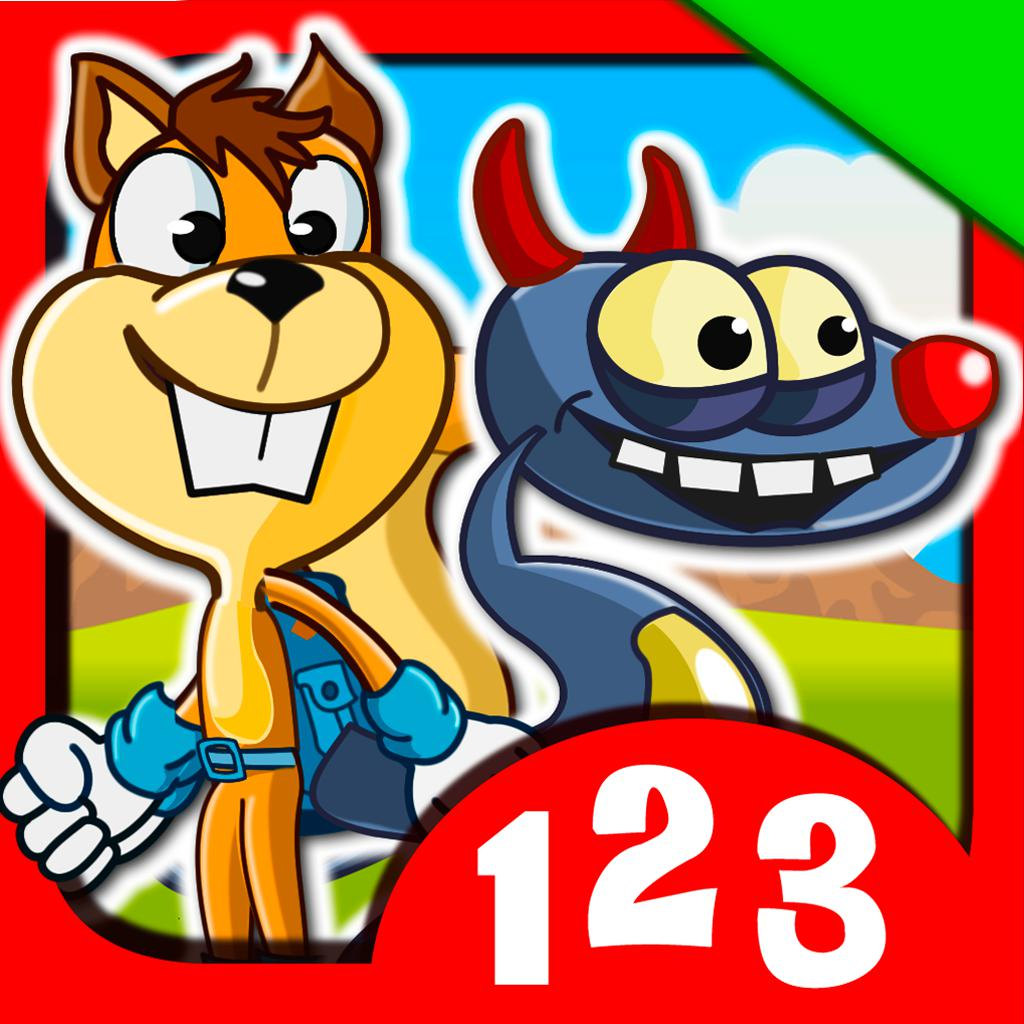Monster Numbers: Basic math-Arithmetic games and addition 4 141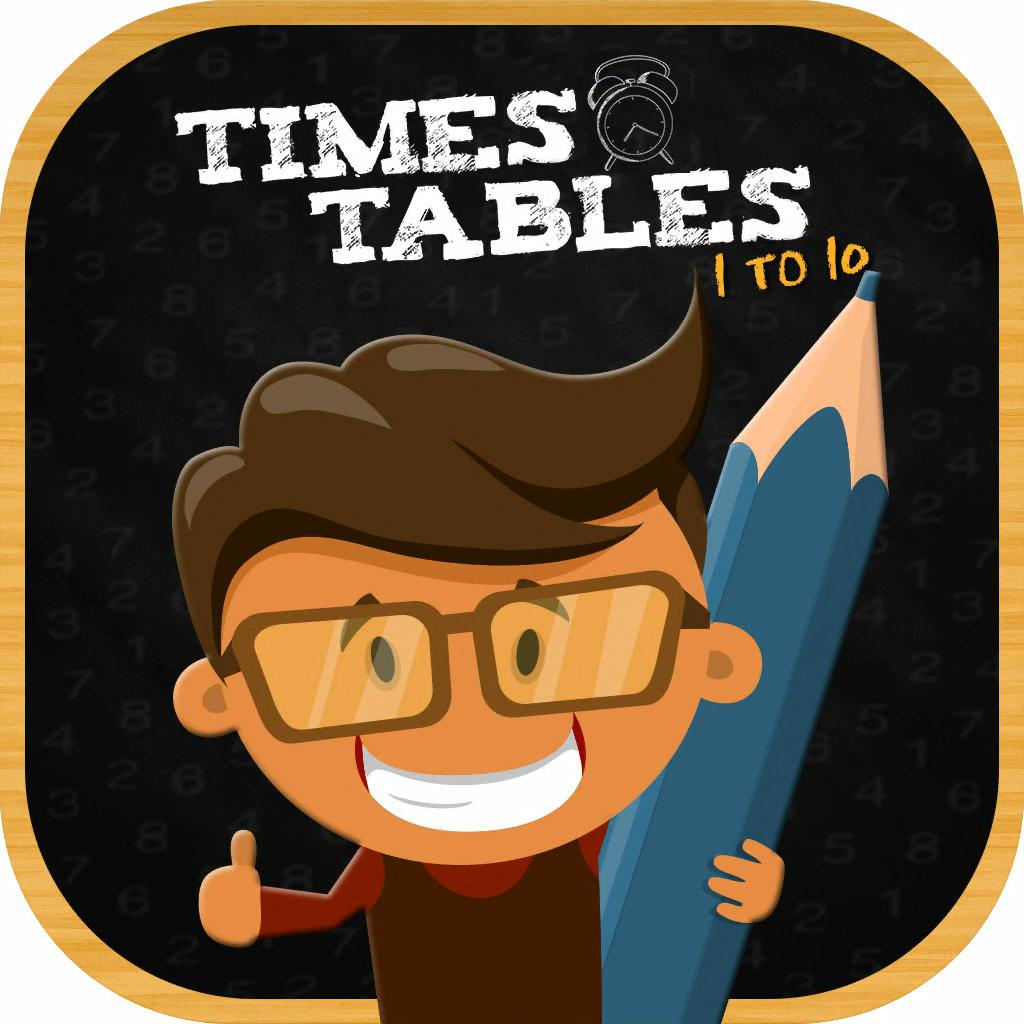Times Tables Multiplication-Learn Math Facts Quiz Games 4 184Zeus vs Monster: Fun Math Game-Mixed Mathematical Operations 4 119

"multiplicand" has 103 related apps in total. Top 5 are Math and Multiplication games, Multiplication Games For Kids., Monster Numbers: Basic math, Times Tables Multiplication, and Zeus vs Monster: Fun Math Game.

• restas y sumas  Related Apps & App Analytics
 Apps Average Star RatingsMath and Multiplication games-Fun 3rd grade math games 4 614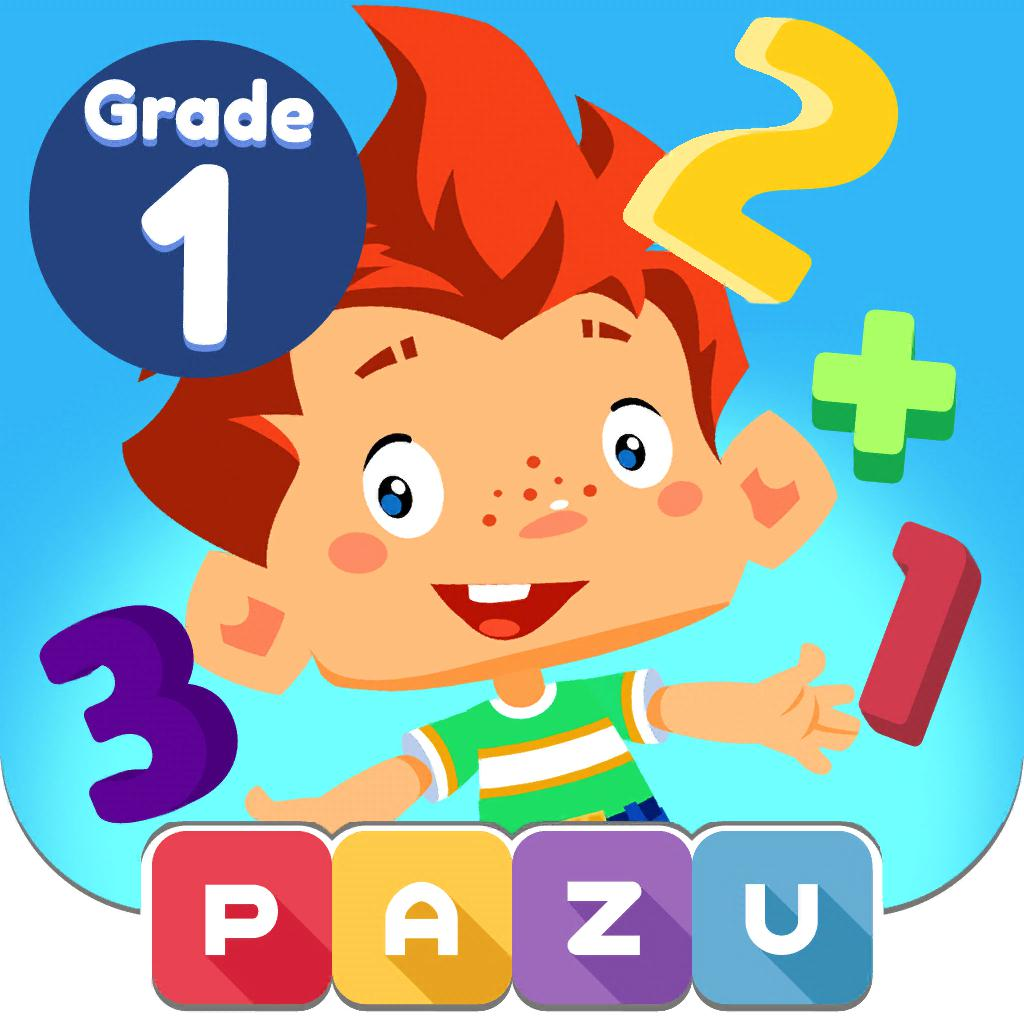Math learning games for kids 1-1st grade addition subtraction 4 293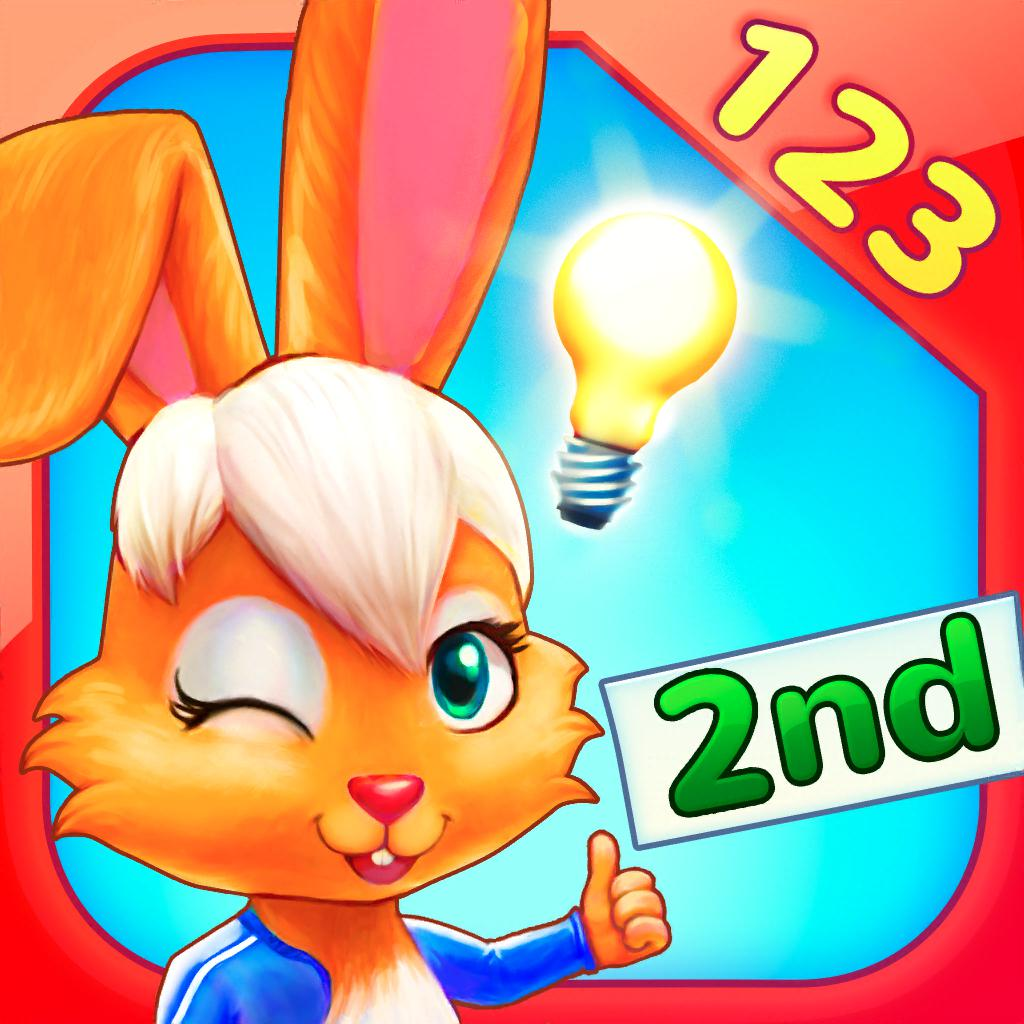Wonder Bunny Math 2nd Gr-Fun Numbers and Counting game 4 63Monster Numbers: Basic math-Arithmetic games and addition 4 141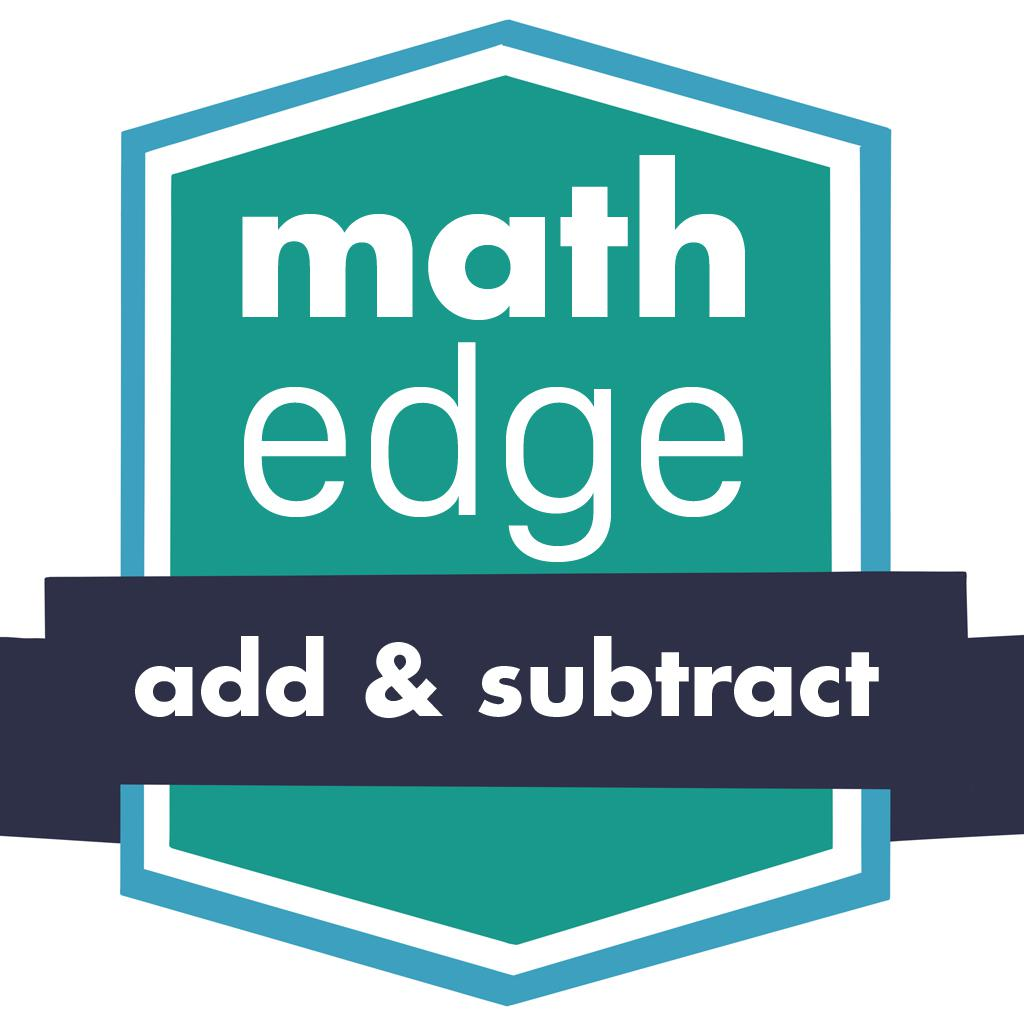MathEdge Add and Subtract-A step-by-step guide to learn! 4 236

"restas y sumas" has 115 related apps in app store. Top 5 are Math and Multiplication games, Math learning games for kids 1, Wonder Bunny Math 2nd Gr, Monster Numbers: Basic math, and MathEdge Add and Subtract.

• juegos de restas y sumas para niños  Related Apps & App Analytics
 Apps Average Star RatingsMath and Multiplication games-Fun 3rd grade math games 4 614Math learning games for kids 1-1st grade addition subtraction 4 293Monster Numbers: Basic math-Arithmetic games and addition 4 141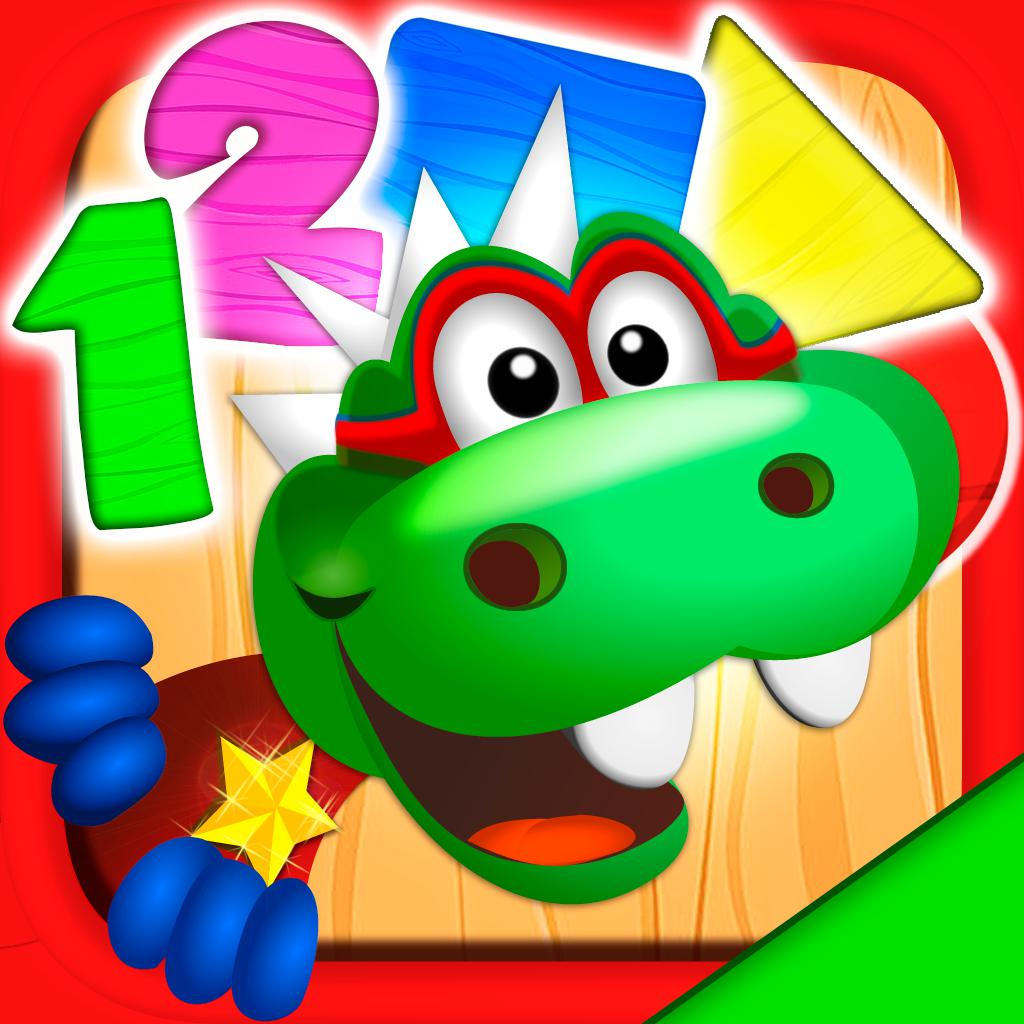Dino Tim: Basic math skills-Learn to count and sequences 4 678Math Land: Arithmetic games-Learn times tables for kids 4 164

"juegos de restas y sumas para niños" has 28 related apps. Top 5 apps are Math and Multiplication games, Math learning games for kids 1, Monster Numbers: Basic math, Dino Tim: Basic math skills, and Math Land: Arithmetic games.

• math planet pro  Related Apps & App Analytics
 Apps Average Star RatingsMath and Multiplication games-Fun 3rd grade math games 4 614Math Rescue: 7－9 Year Old Game-Boys & Girl Educational Games 4 477Kids Learning Tube-Educating kids of all ages 4 308Kids Learning Puzzles: Dogs, My Math Educreations 5 1Animal Fun Facts 5 8

62 apps are ranking for "math planet pro" in app store. Top 5 apps are Math and Multiplication games, Math Rescue: 7－9 Year Old Game, Kids Learning Tube, Kids Learning Puzzles: Dogs, My Math Educreations, and Animal Fun Facts.

As you can see, Math and Multiplication games, Math and Multiplication games, and Math Rescue: 7－9 Year Old Game are ranking for math planet lite, also, Math and Multiplication games, Math and Multiplication games, Multiplication Games For Kids. and Times Tables Multiplication are all competing the keyword multiplicand.

Before promoting your apps in app store, you'd better perform app analytics for better ASO tactics, and ASOTools, free ASO tools will help you with App store keywords optimization.